# RD Sharma Solutions - Chapter 18 - Practical Geometry (Constructions) (Part - 3), Class 8, Maths Notes | Study RD Sharma Solutions for Class 8 Mathematics - Class 8

## Class 8: RD Sharma Solutions - Chapter 18 - Practical Geometry (Constructions) (Part - 3), Class 8, Maths Notes | Study RD Sharma Solutions for Class 8 Mathematics - Class 8

The document RD Sharma Solutions - Chapter 18 - Practical Geometry (Constructions) (Part - 3), Class 8, Maths Notes | Study RD Sharma Solutions for Class 8 Mathematics - Class 8 is a part of the Class 8 Course RD Sharma Solutions for Class 8 Mathematics.
All you need of Class 8 at this link: Class 8

PAGE NO 18.4:

Question 1:

Construct a quadrilateral ABCD in which AB  = 4.4 cm, BC  = 4 cm, CD  = 6.4 cm, DA  = 3.8 cm and BD  = 6.6 cm.

First, we draw a rough sketch of the quadrilateral ABCD and write down its dimensions along the sides.

We may divide the quadrilateral into two constructible triangles ABD and BCD.

Steps of Construction:

Step I: Draw BD = 6.6 cm

Step II: With B as the centre and radius BC = 4 cm, draw an arc.

Step III: With D as the centre and radius 6.4 cm, draw an arc to intersect the arc drawn in Step II at  C.

Step IV: With B as the centre and radius 4.4 cm, draw an arc on the side BD opposite to that of C.

Step V: With D as the centre and radius 3.8 cm, draw an arc to intersect the arc drawn in Step IV at  A.

Step VI: Join BA, DA, BC and CD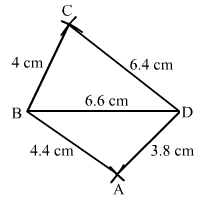Question 2:

Construct a quadrilateral ABCD such that AB  =  BC  = 5.5 cm, CD  = 4 cm, DA  = 6.3 cm and AC  = 9.4 cm. Measure BD.

Steps of construction:

Step I: Draw AB = 5.5 cm

Step II: With B as the centre and radius BC = 5.5 cm, draw an arc.

Step III: With A as the centre and radius AC = 9.4 cm,

draw an arc to intersect the arc drawn in Step II at  C.

Step IV: With C as the centre and radius CD = 4 cm, draw an arc.

Step V: With A as the centre and radius AD = 6.3 cm,

draw an arc to intersect the arc drawn in Step IV at  D.

Step VI: Join DA, BC, AC and CD.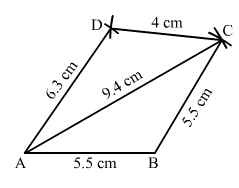Question 3:

Construct a quadrilateral XYZW in which XY  = 5 cm, YZ  = 6 cm, ZW  = 7 cm, WX  = 3 cm and XZ  = 9 cm.

Steps of construction:

Step I: Draw XZ = 9 cm

Step II: With X as the centre and radius 5 cm, draw an arc above XZ.

Step III: With Z as the centre and radius 6 cm, draw an arc to intersect the arc drawn in Step II at Y above XZ.

Step IV: With Z as the centre and radius 7 cm, draw an arc below XZ.

Step V: With X as the centre and radius 3 cm, draw an arc to intersect the arc drawn in Step IV at  W below XZ.

Step VI: Join XY, YZ, ZW and XW.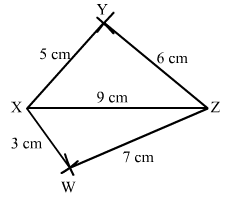Question 4:

Construct a parallelogram PQRS such that PQ  = 5.2 cm, PR  = 6.8 cm and QS  = 8.2 cm.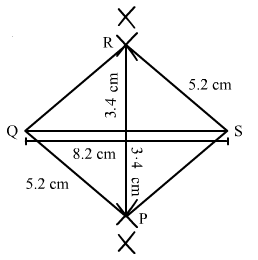In a parallelogram opposite sides are equal.

Thus, we have to construct a quadrilateral PQRS in which

PQ = 5.2 cm,

PR = 6.8 cm and QS = 8.2 cm.

Steps of construction:

Step I: Draw QS = 8.2 cm

Step II: With Q as the centre and radius 5.2 cm, draw an arc.

Step III: With S as the centre and radius 5.2 cm, draw an arc to intersect the arc drawn in Step II at  C.

Step IV: With P as the centre and radius 6.8 cm.

Step V: With Q as the centre and radius 5.2 cm, draw an arc to intersect the arc drawn in Step IV at  A.

Step VI: Join QR, QP, PS and SR.

Question 5:

Construct a rhombus with side 6 cm and one diagonal 8 cm. Measure the other diagonal.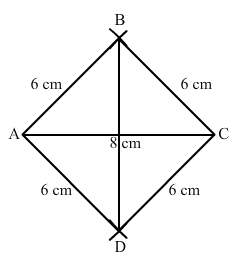Steps of construction:

Step 1: Draw AC = 8 cm.

Step 2: With A as the centre and radius = 6 cm, draw arcs on both sides.

Step 3: With C as the centre and radius = 6 cm,

draw arcs on both sides, intersecting the previous arcs at points B and D.

Step 4: Join BD  = 8.9cm.

Thus, ABCD is the required rhombus.

Question 6:

Construct a kite ABCD in which AB  = 4 cm, BC  = 4.9 cm and AC = 7.2 cm.

Steps of construction:

Step I: Draw AC = 7.2 cm.

Step II: With A as the centre and radius 4cm, draw arcs on both sides of the line segment AC.

Step III : With C as the centre and radius 4.9 cm, draw arcs on both sides of AC intersecting the previous arcs of step II at B and D.

Step IV: Join BA, DA, BC and CD.

Thus, the quadrilateral ABCD so obtained is the required kite.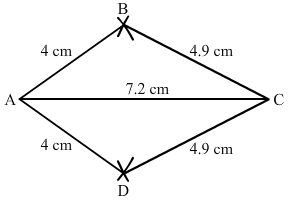Question 7:

Construct, if possible, a quadrilateral ABCD given AB  = 6 cm, BC  = 3.7 cm, CD  = 5.7 cm, AD  = 5.5 cm and BD  = 6.1 cm. Give reasons for not being able to construct it, if you cannot.

Steps of construction:

Step I: Draw AB = 6 cm.

Step II: With A as the centre and radius 5.5 cm, draw an arc.

Step III : With B as the centre and radius 6.1 cm, draw an arc to intersect the arc drawn in StepII at D.

Step IV: With B as the centre and radius 3.7 cm, draw an arc on the side.

Step V :With D as the centre and radius 5.7 cm, draw an arc to intersect the arc drawn in StepIV at C.

Step VI: Join BD, DA, BC and CD.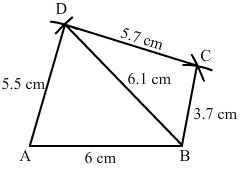Question 8:

Construct, if possible, a quadrilateral ABCD in which AB  = 6 cm, BC  = 7 cm, CD  = 3 cm, AD  = 5.5 cm and AC  = 11 cm. Give reasons for not being able to construct, if you cannot. (Not possible, because in triangle ACD, AD + CD < AC).

Such a quadrilateral cannot be constructed because in a triangle, the sum of the length of its two sides must be greater than the that of the third side
But here in triangle ACD,
AD + CD = 5.5 + 3 = 8.5 cm
and AC = 11 cm
i.e., AD + CD <  AC, which is not possible.
So, the construction is not possible.

The document RD Sharma Solutions - Chapter 18 - Practical Geometry (Constructions) (Part - 3), Class 8, Maths Notes | Study RD Sharma Solutions for Class 8 Mathematics - Class 8 is a part of the Class 8 Course RD Sharma Solutions for Class 8 Mathematics.
All you need of Class 8 at this link: Class 8Use Code STAYHOME200 and get INR 200 additional OFF

## RD Sharma Solutions for Class 8 Mathematics

88 docs

Track your progress, build streaks, highlight & save important lessons and more!

,

,

,

,

,

,

,

,

,

,

,

,

,

,

,

,

,

,

,

,

,

,

,

,

,

,

,

;